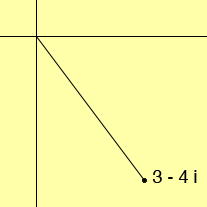SEARCH HOMEMath Central Quandaries & QueriesQuestion from roland: write the given complex number z in polar form lzl(p+qi) where lp + qil=1 for 3 - 4i.Hi Roland,

First use Pythagoras' Theorem to find the |z|, the modulus of z where z = 3 - 4i and then

z = |z| (3/|z| - 4/|z| i)

so p + q i = 3/|z| - 4/|z| i

What remains is to find the argument of z. Since 3 - 4 i is in the 4th quadrant the argument must be between 270 and 360 degrees. Use this fact and he inverse tangent function to determine the argument of z.HarleyMath Central is supported by the University of Regina and The Pacific Institute for the Mathematical Sciences.